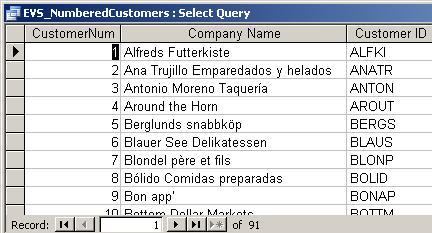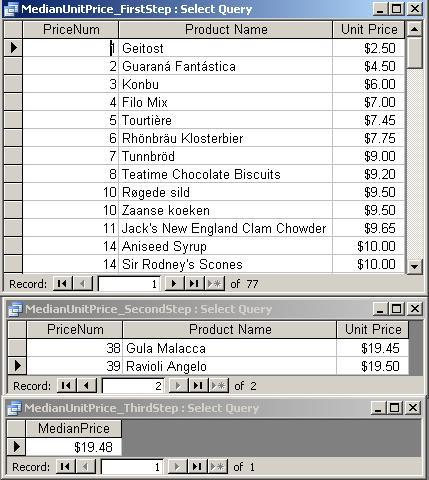STATISTICS IN SQL

Note: Can be done in the database, but best done in a spreadsheet or using statistics software!

EXAMPLES

• Mean:  AVG (aggregate function)
•  Mode:  Count of most frequently occurring value  (combination of aggregate functions and subquery)
E.g.: Price frequency (upper result) and mode (lower result)SQL for the two queries:

SELECT Products.UnitPrice, Count(*) AS Frequency
FROM Products
GROUP BY Products.UnitPrice
ORDER BY Count(*) DESC , UnitPrice;

SELECT UnitPrice, Count(*) AS Frequency
FROM Products
GROUP BY UnitPrice
HAVING Count(*) >= ALL
(SELECT Count(*)
FROM Products
GROUP BY UnitPrice);

Note:  We need the subquery because aggregate functions cannot be nested.

• Median:   Value such that the number of values above and below it is the same ("middle" value, not necessarily same as average or mean).  (Moved after running count and running sums because it requires a running count and is more complex than either.)
• Ranking; running count: Compare table to itself (self-join) and generate count based on the comparison.
E.g.:  Assign sequential numbers to customers based on their customer ID.Note:  CustomerNum is not stored in the database - it is generated whenever needed by running the query.
SQL for the query:

SELECT count(p1.CustomerID) AS CustomerNum, p1.CompanyName,  p1.CustomerID
FROM Customers  p1
INNER JOIN Customers p2
ON p1.CustomerID >= p2.CustomerID
GROUP BY p1.CompanyName, p1.CustomerID
ORDER BY 1;

•  Running sum:  Compare table to itself (self-join) and generate totals based on the comparison.
E.g.:  Calculate the running total \$ amount by date for orders placed by a particular customer (ID RATTC). To simplify the SQL, first calculate total for this customer without generating a running total and then the running total based on the first query (results below, on the left):The result on the right includes a running count based on date as well as the running total.

SQL for the three queries:

Query at top left (RATTC_ForRunSum)

SELECT a.OrderID, a.OrderDate, Sum(a.ExtendedPrice) AS OrderTotal
FROM Invoices AS a
WHERE a.CustomerID = "RATTC"
GROUP BY a.OrderID, a.OrderDate;

Query at lower left with running total only (RATTC_RunningTotal):

SELECT a.OrderID, a.OrderDate, Sum(b.OrderTotal) AS RunningTotal
FROM RATTC_ForRunSum AS a, RATTC_ForRunSum AS b
WHERE b.OrderDate <= a.OrderDate
GROUP BY a.OrderID, a.OrderDate;

Query at lower right with running total and count (RATTC_RunningTotalAndCount):

SELECT Count(b.OrderDate) AS DateCount, a.OrderID, a.OrderDate, Sum(b.OrderTotal) AS RunningTotal
FROM RATTC_ForRunSum AS a, RATTC_ForRunSum AS b
WHERE b.OrderDate <= a.OrderDate
GROUP BY a.OrderID, a.OrderDate;

• Median:   Value such that the number of values above and below it is the same ("middle" value, not necessarily same as average or mean).  The solution offered here is an approximation - may not work in all cases.  Also, other database systems have better functions for finding the "middle" of a sorted list.

E.g.:  Find the median unit price for all products.  Build the query in three steps (results below the third SQL statement):

• Number the product list based on UnitPrice:
SELECT Count(a.ProductID) AS PriceNum, a.ProductName, a.UnitPrice
FROM Products AS a
INNER JOIN Products AS b
ON a.UnitPrice>=b.UnitPrice
GROUP BY a.ProductID, a.ProductName, a.UnitPrice
ORDER BY a.UnitPrice;

• Find the middle one or two numbers (added clauses highlighted):

SELECT Count(a.ProductID) AS PriceNum, a.ProductName, a.UnitPrice
FROM Products AS a
INNER JOIN Products AS b
ON a.UnitPrice >= b.UnitPrice
GROUP BY a.ProductID, a.ProductName, a.UnitPrice
HAVING Count(a.ProductID)
Between (SELECT (Count(ProductID)/2)-0.5
FROM Products)
And        (SELECT (Count(a.ProductID)/2)+0.5
FROM Products)
ORDER BY a.UnitPrice;

• Average the results (added clauses highlighted):

SELECT Avg(UnitPrice) AS MedianPrice
FROM
(SELECT Count(a.ProductID) AS PriceNum, a.ProductName, a.UnitPrice
FROM Products AS a
INNER JOIN Products AS b
ON a.UnitPrice>=b.UnitPrice
GROUP BY a.ProductID, a.ProductName, a.UnitPrice
HAVING Count(a.ProductID)
Between
(SELECT (Count(ProductID)/2)-0.5
FROM Products)
And
(SELECT (Count(a.ProductID)/2)+0.5
FROM Products));